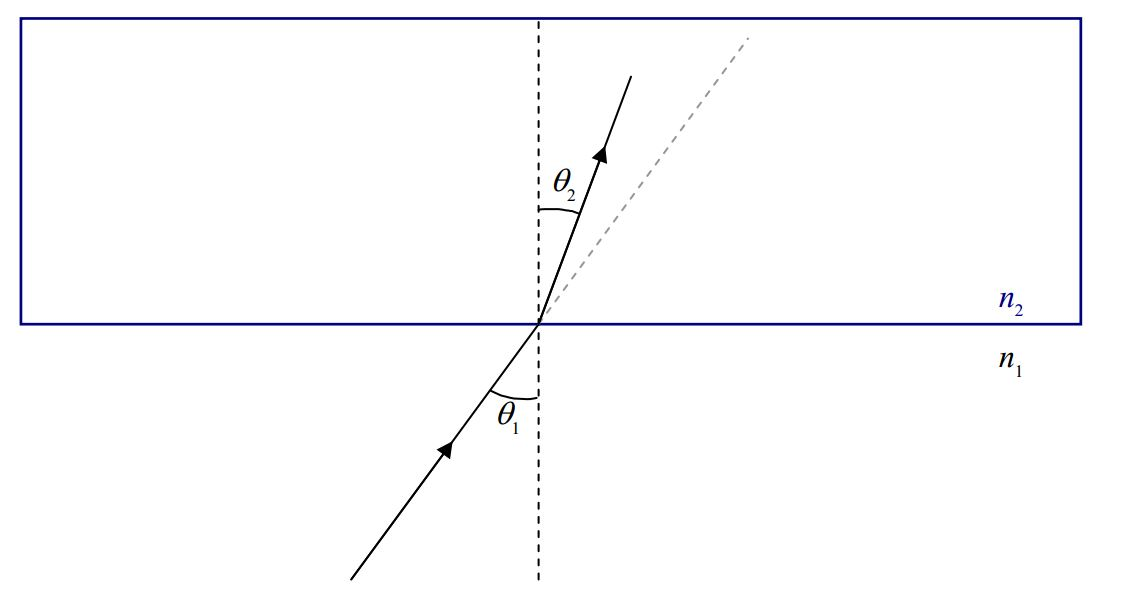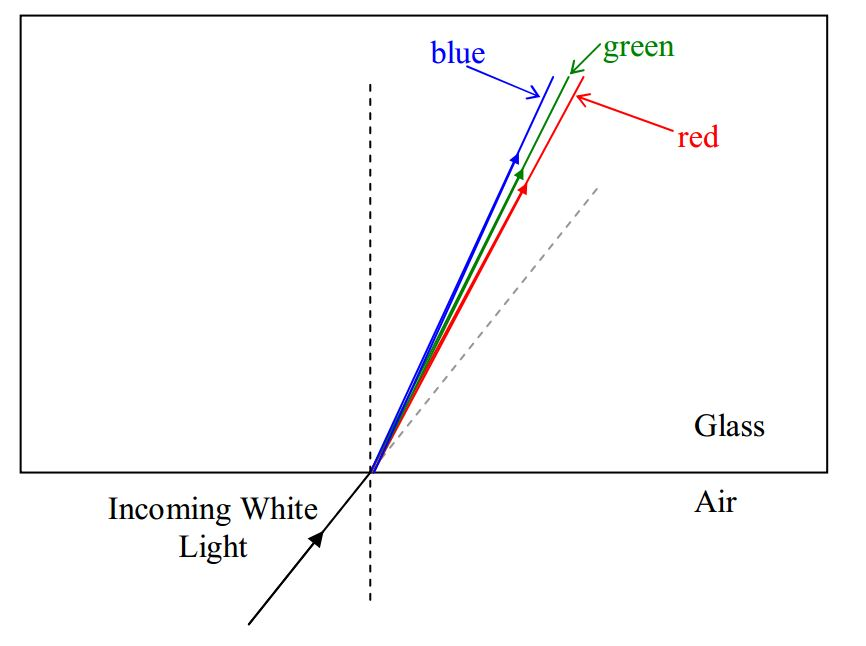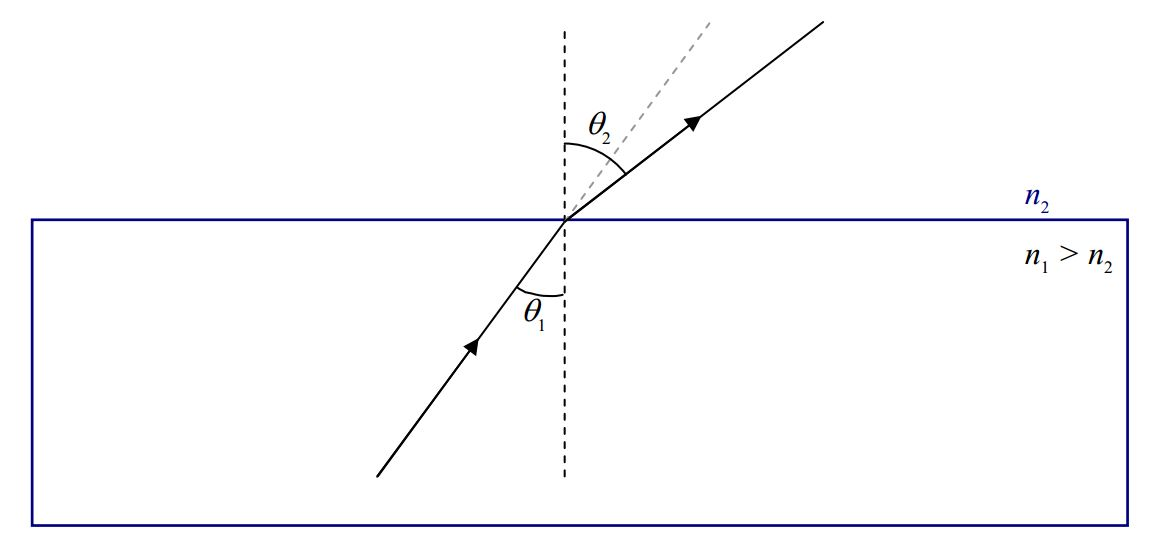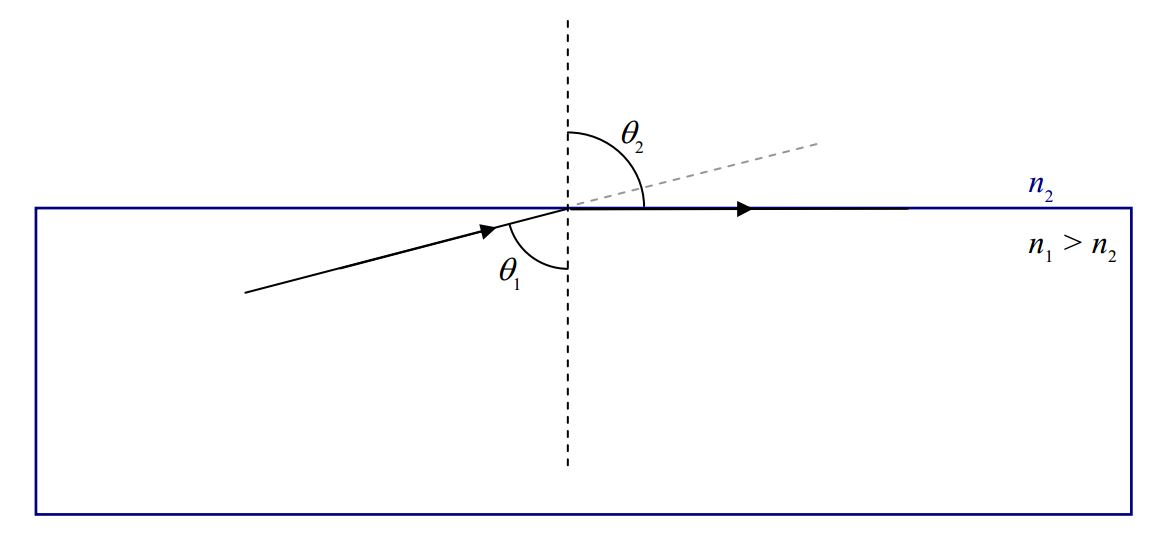$$\require{cancel}$$

# B27: Refraction, Dispersion, Internal Reflection

When we talked about thin film interference, we said that when light encounters a smooth interface between two transparent media, some of the light gets through, and some bounces off. There we limited the discussion to the case of normal incidence. (Recall that normal means perpendicular to and normal incidence is the case where the direction in which the light is traveling is perpendicular to the interface.) Now we consider the case in which light shining on the smooth interface between two transparent media, is not normally incident upon the interface. Here’s a “clean” depiction of what I’m talking about:and here’s one that’s all cluttered up with labels providing terminology that you need to know:As in the case of normal incidence, some of the light is reflected and some of it is transmitted through the interface. Here we focus our attention on the light that gets through.Experimentally we find that the light that gets through travels along a different straight line path than the one along which the incoming ray travels. As such, the transmitted ray makes an angle $$\theta_2$$ with the normal that is different from the angle $$\theta_1$$ that the incident ray makes with the normal.

The adoption of a new path by the transmitted ray, at the interface between two transparent media is referred to as refraction. The transmitted ray is typically referred to as the refracted ray, and the angle $$\theta_2$$ that the refracted ray makes with the normal is called the angle of refraction. Experimentally, we find that the angle of refraction $$\theta_2$$ is related to the angle of incidence $$\theta_1$$ by Snell’s Law:

$n_1 \sin\theta_1=n_2 \sin\theta_2 \label{27-1}$

where:

• $$n_1$$ is the index of refraction of the first medium, the medium in which the light is traveling before it gets to the interface,
• $$\theta_1$$ is the angle that the incident ray (the ray in the first medium) makes with the normal,
• $$n_2$$ is the index of refraction of the second medium, the medium in which the light is traveling after it goes through the interface, and,
• $$\theta_2$$ is the angle that the refracted ray (the ray in the second medium) makes with the normal.

# Dispersion

On each side of the equation form of Snell’s law we have an index of refraction. The index of refraction has the same meaning as it did when we talked about it in the context of thin film interference. It applies to a given medium. It is the ratio of the speed of light in that medium to the speed of light in vacuum. At that time, I mentioned that different materials have different indices of refraction, and in fact, provided you with the following table:

Medium Index of Refraction
Vacuum 1
Air 1.00
Water 1.33
Glass(Depends on the kind of glass. Here is one typical value.) 1.5

What I didn’t mention back then is that there is a slight dependence of the index of refraction on the wavelength of the visible light, such that, the shorter the wavelength of the light, the greater the index of refraction. For instance, a particular kind of glass might have an index of refraction of 1.49 for light of wavelength 695 nm (red light), but an index of refraction that is greater than that for shorter wavelengths, including an index of refraction of 1.51 for light of wavelength 405 nm (blue light). The effect in the case of a ray of white light traveling in air and encountering an interface between air and glass is to cause the different wavelengths of the light making up the white light to refract at different angles.This phenomena of white light being separated into its constituent wavelengths because of the dependence of the index of refraction on wavelength, is called dispersion.

# Total Internal Reflection

In the case where the index of refraction of the first medium is greater than the index of refraction of the second medium, the angle of refraction is greater than the angle of incidence.For such a case, look what happens when we increase the angle of incidence, $$\theta_1$$:The angle of refraction gets bigger… until eventually it (the angle of refraction) gets to be $$90^{\circ}$$.It can’t get any bigger than that, because, beyond that, the light is not going through the interface. An angle of refraction greater than $$90^{\circ}$$ has no meaning. But, note that we still have room to increase the angle of incidence. What happens if we continue to increase the angle of incidence? Well, indeed, no light gets through the interface. But, remember at the beginning of this chapter where we talked about how, when light is incident on the interface between two transparent media, some of the light gets through and some of it is reflected? Well, I haven’t been including the reflected ray on our diagrams because we have been focusing our attention on the transmitted ray, but, it is always there. The thing is, at angles of incidence bigger then the angle that makes the angle of refraction $$90^{\circ}$$, the reflected ray is all there is. The phenomenon, in which there is no transmitted light at all, just reflected light, is known as total internal reflection. The angle of incidence that makes the angle of refraction $$90^{\circ}$$ is known as the critical angle. At any angle of incidence greater than the critical angle, the light experiences total internal reflection. Note that the phenomenon of total internal reflection only occurs when the light is initially in the medium with the bigger index of refraction.

Let’s investigate this phenomenon mathematically. Starting with Snell’s Law:

$n_1 \sin\theta_1=n_2 \sin \theta_2$

solved for the sine of the angle of refraction:

$\sin \theta_2 =\frac{n_1}{n_2} \sin\theta_1$

we note that, since it was stipulated that $$n_1>n_2$$, the ratio $$n_1/n_2$$ is greater than 1. The $$\sin \theta_1$$ is always less than $$1$$, but, if $$\theta_1$$ is big enough, $$\sin\theta_1$$ can be so close to $$1$$ that the right-hand side of the equation $$\sin \theta_2=\frac{n_1}{n_2} \sin\theta_1$$ is greater than $$1$$. In that case, there is no $$\theta_2$$ that will solve the equation because there is no angle whose sine is greater than 1. This is consistent with the experimental fact that, at angles of incidence greater than the critical angle, no light gets through the interface. Let’s solve for the critical angle. At the critical angle, the angle of refraction $$\theta_2$$ is $$90^{\circ}$$. Let’s plug that into the equation we have been working with and solve for $$\theta_1$$:

$\sin \theta_2=\frac{n_1}{n_2} \sin\theta_1$

evaluated at $$\theta_2 = 90^{\circ}$$ yields:

$\sin 90^{\circ}=\frac{n_1}{n_2} \sin\theta_1$

$1=\frac{n_1}{n_2} \sin\theta_1$

$\sin \theta_1=\frac{n_2}{n_1}$

$\theta_1= \sin^{-1} \frac{n_2}{n_1}$

This is such a special angle of incidence that we not only give it a name (as mentioned, it is called the critical angle), but, we give it its own symbol. The critical angle, that angle of incidence beyond which there is no transmitted light, is designated $$\theta_C$$ , and, as we just found, can be expressed as:

$\theta_C=\sin ^{-1} \frac{n_2}{n_1} \label{27-2}$